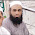# Basics of Electrical Engineering

Learn the basics of Electrical Engineering.

While discussing basic of Electrical Engineering one of the most commonly discussed question is "What kills: ac or dc". After reading this post you'll be able to answer the question. Things you'll learn:

1. How current flows
2. What's the resistance of human body

### How current flows?

Current is the flow of electrical charges which move from higher potential to the lower potential. The actual amount of current flowing through a body depends on two factors:
1. Applied voltage
2. Resistance of body
Let's consider that you are a resistor (Just for a moment 😀) whose value is 1 kΩ. Accidently you touch the live terminal having a value 120 V. A current of 120 mA will flow through your body.
Let's consider another case where I am a resistor whose value is 500 kΩ. In my case, a current of  0.24 mA will flow through me. Now let's roll out of the comparative world.

It's actually the electrical current which damages the human body. A current of 120 mA is too much dangerous whereas a current of 0.24 mA is comparatively very small to cause damage.

Summarizing above all it is the current which actually kills the human body, but it is the voltage which creates this current and finally, it is the resistance which controls the flow of current.

### What's the resistance of human body?

The actual resistance of our body depends on moisture content. A wet skin has 1,000 Ω, whereas a dry skin offers high resistance as 50 kΩ - 500 kΩ. These are quite high values which provide a safe signal in most cases.

However, the internal resistance of body is 500 Ω. Any sort of cut on our body reduces 50 k to 500 Ω. So, you should be extremely careful in such circumstances.

Final words:
What kills you?
Short answer: It is the current.
Long answer: It is current, but this current depends on the voltage and resistance.

1.2.